Implementation of the WEIGHT Statement

You can use the WEIGHT statement to specify a weight variable in the input data set. See the section WEIGHT Statement for more information. This section describes how PROC ROBUSTREG implements the WEIGHT statement for each of the estimation methods and for leverage detection.

M Estimation

If you use M estimation with a known scale, instead of minimizing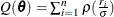, the weighted M estimation minimizes the weighted Huber-type objective function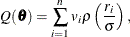where v is the weight variable specified by the WEIGHT statement. If you use M estimation with an unknown scale, the weight variable is used in the location steps but not in the scale steps. See the section M Estimation and the SCALE= option for more details. For estimating the covariance of the weighted M estimation,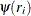and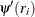are obtained from the final iteration of the weighted M estimation, and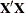and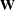are respectively replaced by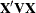and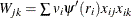, where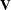is a diagonal matrix with its diagonal elements being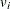’s. See the section Asymptotic Covariance and Confidence Intervals for more information. The weight variable does not affect the model degrees of freedom p and the error degrees of freedom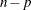.

LTS Estimation

LTS estimation ignores the weight variable.

S Estimation

S estimation applies the weight variable only in its M-refinement step. Except for the initial estimates, the M-refinement step of S estimation is the same as the weighted M estimation with unknown scale. If you use the NOREFINE suboption, S estimation ignores the weight variable along with the M-refinement step.

MM Estimation

By default, the initial step of MM estimation is the initial LTS estimation. Unlike the regular LTS estimation, the initial LTS estimation is applied on the weighted data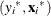’s, where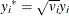and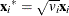. After the initial LTS estimation, the weight variable is ignored for the subsequent scale adjustment.

You can use INITEST=S to specify the initial S estimation as the initial step of the MM estimation. Similarly to the regular S estimation, the weight variable is used only in the M-refinement step of the initial S estimation. There is no subsequent scale adjustment step if the initial S estimation is applied.

Except for the initial estimates, the final M estimation of the MM estimation is the same as the weighted M estimation with known scale.

Final Weighted Least Squares Estimation

Final weighted least squares estimation is always applied on the weighted datano matter how the weight variable is applied in the preceding estimation. For example, if the option METHOD=LTS is specified along with the option FWLS, although the outliers identified by LTS estimation do not depend on the weight variable, final weighted least squares estimation applies the weight variable on all the points that are not outliers.

Robust Distances and Leverage Detection

Robust distance computation ignores the weight variable. Because leverage detection depends on robust distance, it also ignores the weight variable.